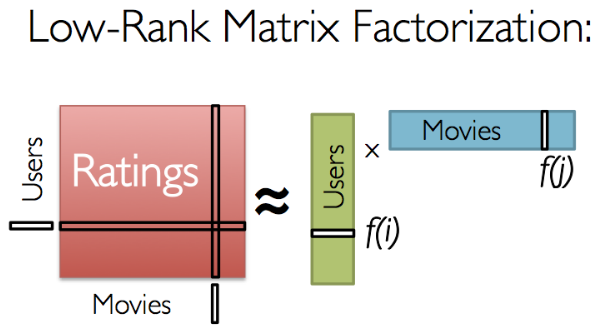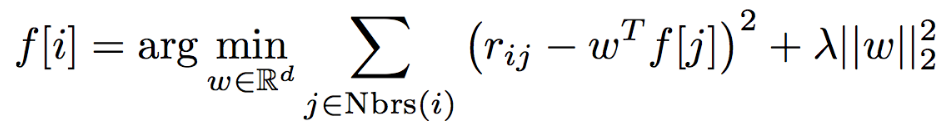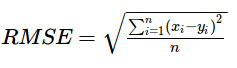[TOC]

# Part 0: Preliminaries

Each line in the ratings dataset (ratings.dat.gz) is formatted as:
`UserID::MovieID::Rating::Timestamp` ⇒ tuples of `(UserID, MovieID, Rating)`in ratingsRDD
Each line in the movies (movies.dat) dataset is formatted as:
`MovieID::Title::Genres` ⇒ tuples of `(MovieID, Title)` in ratingsRDD

487650 ratings and 3883 movies

⇒ Since the key is an integer and the value is a unicode string, we can use a function to combine them into a single unicode string (e.g., `unicode('%.3f' % key) + ' ' + value`) before sorting the RDD using `sortBy()`.

# Part 1: Basic Recommendations

naive method: always recommend the movies with the highest average rating... ⇒ 20 movies with the highest average rating and more than 500 reviews

movieNameWithAvgRatingsRDD: `(avgRating, Title, nbRatings)`

# Part 2: Collaborative Filtering

Collaborative filtering is a method of making automatic predictions (filtering) about the interests of a user by collecting preferences or taste information from many users (collaborating). The underlying assumption of the collaborative filtering approach is that if a person A has the same opinion as a person B on an issue, A is more likely to have B's opinion on a different issue x than to have the opinion on x of a person chosen randomly.### Matrix Factorization

CF问题实际上是矩阵分解的问题: We have a matrix whose entries are movie ratings by users (shown in red in the diagram below). Each column represents a user (shown in green) and each row represents a particular movie (shown in blue).

With collaborative filtering, the idea is to approximate the ratings matrix by factorizing it as the product of two matrices: one that describes properties of each user (shown in green), and one that describes properties of each movie (shown in blue).(后面加上的那一项是正则项: 不希望f[i]的模过大)

first randomly filling the users matrix with values and then optimizing the value of the movies such that the error is minimized. Then, it holds the movies matrix constrant and optimizes the value of the user's matrix.

### train-test-validation split

⇒ break up the ratingsRDD dataset into three pieces:

• A training set (RDD), which we will use to train models
• A validation set (RDD), which we will use to choose the best model
• A test set (RDD), which we will use for our experiments

`trainingRDD, validationRDD, testRDD = ratingsRDD.randomSplit([6, 2, 2], seed=0L)`

### Root Mean Square Error (RMSE)compute the sum of squared error given predictedRDD and actualRDD RDDs. Both RDDs consist of tuples of the form (UserID, MovieID, Rating)

### alternating least square of MLllib

https://spark.apache.org/docs/latest/api/python/pyspark.mllib.html#pyspark.mllib.recommendation.ALS ALS takes a training dataset (RDD) and several parameters that control the model creation process.

The most important parameter to ALS.train() is the rank, which is the number of rows in the Users matrix (green in the diagram above) or the number of columns in the Movies matrix (blue in the diagram above). (In general, a lower rank will mean higher error on the training dataset, but a high rank may lead to overfitting.)

```model = ALS.train(trainingRDD, rank, seed=seed, iterations=iterations,
lambda_=regularizationParameter)
predictedRatingsRDD = model.predictAll(validationForPredictRDD)
```

### compare model

Looking at the RMSE for the results predicted by the model versus the values in the test set is one way to evalute the quality of our model. Another way to evaluate the model is to evaluate the error from a test set where every rating is the average rating for the training set. ⇒ 这里没有太理解, 难道是说test set 的平均rating预测结果和training set的平均rating应该比较接近么?? ⇒ 终于明白了: "Your model more accurately predicts the ratings than using just the average rating, as the model's RMSE is significantly lower than the RMSE when using the average rating."

• 求一个tuple rdd最后一列的和的时候, 需要先map成最后一列再reduce:

`trainingRDD.map(lambda x:x[-1]).reduce(lambda x,y:x+y)`

#### Part 6 of series «spark MOOC»：

comments powered by Disqus